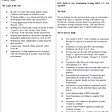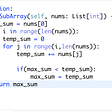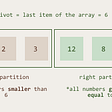# Algorithms and how to analyze their complexity

Algorithms are involved in nearly all the tasks we perform on a daily basis. We execute many of them on “automatic”, without even realizing “what” they are used for or “how” we use them. In software development, it’s not that different. But, what are algorithms anyways? And why is it important to analyze their complexity?

Let’s find out! :)

# What is an algorithm?

A set of steps necessary to perform a task.

Computer programs aren’t the only things that execute algorithms, they’re also executed and implemented by us humans.

For example, have you thought of the algorithm that executes the following tasks?

• Take a bath
• Cook
• Drive to work from home

In our day-to-day, we execute a series of steps to complete at least one of the tasks mentioned above. Let’s use the task driving to work from home as an example. The steps I take are basically as follows:

1. Get in my car
2. Drive to a friend’s house to give them a ride
3. Drive to the office where I work
4. Park my car in the parking garage

This is the set of steps (algorithm) I need to perform to complete this task.

Now, think about the necessary set of steps you take to complete the same task. It’s likely that they’re somewhat different from mine. Our algorithms might be different, but our objective is the same.

Computer programs aren’t too different: there are a variety of algorithms that can be used to carry out a series of tasks. Often, we don’t concern ourselves with what they are, we simply use them (myself included).

For example, what should the algorithm used by Waze and Google Maps, the one that calculates the best route between two locations, look like? What about Netflix’s algorithm that suggests movies and series based on what you’ve watched?

Personally, I couldn’t tell you. However, I do know that they’re efficient. And efficiency is the standard we use to analyze the complexity of an algorithm.

# What is Algorithm Complexity?

In computer science, algorithm complexity refers to how much time and space an algorithm consumes to execute a task, according to the size of its input.

Generally, algorithms are evaluated by their consumption of both time and space, but I’m only going to address time complexity in this post.

Analyzing time complexity allows us to determine how an algorithm will behave given an increase in its input elements. We can have an idea of the time it would take to process 10, 100, or 1000 elements.

And why is this analysis important?

• To determine which algorithm is most efficient;
• To be able to develop more efficient algorithms;
• To be able to analyze whether an algorithm’s library, or even it’s actual language, are efficient.

To summarize, efficiency is the point!

# Time Complexity

The time it takes for an activity to conclude.

We can begin analyzing time with the Frequency Count method, which basically counts the number of times a machine instruction is executed. Each step (instruction) is assigned a time unit, starting with 1.

The time that the algorithm consumes is expressed by the funcion f(n), where n represents the data size.

The result of an analysis is expressed as follows:

([function that expresses time]) = [Sum of the time units]

Now let’s analyze some algorithms to understand how this works in reality.

The first function adds up two whole numbers:

We can see that, for said task, the implemented algorithm only executes a single step: the summing of two numbers. Since we attribute a value for each step, the result is f(n) = 1.

Let’s look at the next example, which is an algorithm that divides an integer by another integer:

Despite being a mathematical operation similar to summation, the algorithm contains more steps because it needs to check if the divisor is 0; if this is the case, the division won’t be realized. Since we have four steps in total, and each of these is assigned a value of 1, the result is f(n) = 4.

The next algorithm sums up all the elements of a list of integers:

In this algorithm, one of the steps includes for, an instruction for repetition; this means that the code inside of for will be executed several times. Since the number of executions depends on the size of the data, we attribute the value of n as a time unit. The result is f(n) = 2n + 3.

The next algorithm adds the sum of one list with the sum of a second list.

As a result we have f(n) = 2n² + 2n + 3.

Up to this point we’ve only seen simple algorithms, right? Now imagine analyzing more complex algorithms and needing to perform these calculations? Not very feasible, is it? Although it seems the most appropriate, it is very laborious and, at the end of the day, isn’t worth it.

Usually, when we analyze an algorithm, we are trying to find out its behaviors when there are many elements to process. It’s in these situations that we need to find the predominant term of the sum of the time units.

For example, what’s the predominant term of the sum of the third algorithm?

f(n) = 2n + 3

In this case, the predominant term in 2*n, and I’ll explain why!

In any situation where n is greater than 1 (n > 1), the product (the result of multiplication) will already be worth more than the second term of the sum.

Test something: substitute n with any integer greater than 1, and perform the multiplication.

What about the predominant term of the sum of the last algorithm?

f(n) = 2n² + 2n + 3

In this case, the predominant term is 2*n², because when n > 1, the product will always be greater than the second and third terms of the sum. Go ahead, test it!

Ergo, there is a more common way, so to speak, to analyze the complexity of algorithms, where constants and less significant terms are disregarded. This method is called Asymptotic Complexity.

This is where the Order of Complexity comes into play, also known as Notation-O or Big-O.

# Big-O Notation

Used to classify an algorithm considering the growth rate of operations as the number of processed elements grows.

Big-O notation also defines a function which expresses the time complexity of an algorithm. To this end, n is used as a function of the letter O.

The most common classes are:

• O(1) — Constant, number of operations doesn’t increase as the number of elements increases
• O(log n) — Logarithmic, number of operations is less than the number of elements
• O(n) — Linear, the number of operations increases proportionally with the number of elements
• O(n²) Quadratic, the number of operations will be greater than the number of elements
• O(2^n) — Exponencial, the number of operations increases rapidly compared to the number of elements
• O(n!) — Factorial, the number of operations increases very, very rapidly, even for a small number of elements

Below, we have a graphic which illustrates the Growth Rate of Operations x Quantity of Elements:

The graphic is classified as follows:

• Red zone is terrible, avoid it!
• Orange zone is bad, also avoid it!
• Yellow zone is fair, reasonable!
• Light-green zone is good, cool!
• Dark-green zone is excellent, congrats!

Now, we’re going to use Big-O notation to categorize the algorithms that were previously mentioned, always using their predominant term to guide us.

The first algorithm resulted in f(n) = 1; meaning that, independent of an increase in elements, the algorithm only executes one operation. Thus, we can classify the algorithm as Constant — O(1).

The second algorithm resulted in f(n) = 4; meaning that, independent of an increase in elements, the algorithm will execute four operations. Thus, we can also classify this algorithm as Constant — O(1).

The result of the third algorithm was f(n) = 2n + 3; meaning that the number of operations will grow proportionally with the number of elements, seeing as how n serves as a variable in this function. Thus, we can define this algorithm as Linear — O(n).

The result of the last algorithm resulted in f(n) = 2n² + 2n + 3, meaning that the number of operations will increase more than the number of elements. In this case, n also serves as a variable, but it is raised to the second power (squared). Thus, we can define this algorithm as Quadratic — O(n²).

The table below illustrates the growth in the number of operations as it relates to the growth of the number of elements.

Notice that in an exponential algorithm, 16 elements would result in at least 65,5536 operations. Pretty disturbing, right?

In general, the analysis process is the same as we’ve been doing: counting the number of steps and identifying the predominant term.

Some trends we can observe:

• Algorithms that don’t have a repetition loop tend to be Constant — O(1)
• Algorithms that have repetition loops, as long as they’re not nested, tend to be Linear — O(n)
• Algorithms that have two nested repetition loops tend to be Quadratic — O(n²)
• Direct access to the index of an array is usually O(1) (constant)
• List extension methods, on average, are O(n). For example: any, sum, and filter.
• Sorting algorithms like Bubble Sort, Insertion Sort, and Selection Sort are usually O(n²).

Knowing how to classify algorithms gives us the capacity to judge an algorithm’s efficiency or inefficiency. Of course, we can’t measure its exact running time in seconds, minutes, or hours. To do this, it needs to be executed, measured, and altered accordingly. Imagine doing this for algorithms that take hours, or even days, to run? Not a chance!

I hope I have fulfilled the goal of this post, which was to give an overview of algorithms and their analysis using the Frequency Count and Big-O methods.

References:

Vídeos 1 to 1.7

Want to bring innovation to the loan market through technology? We’re always looking for new people to join our crew!

Check out our openings here..

--

--

--

## More from Creditas Tech

Our technologies, innovations, digital product management, culture, and much more!

## Sharpen the competitive edge of your IT: move your code to containers## Today's Role of a QA Engineer## You Should Learn Rails## Array in python## Enterprise Software Patterns: Part 2## Building an ideal Azure SQL Server and the database using ARM templates in minutes## Algorithm Test Engineering: Exploratory Job Analysis## Data Structure Problem | Maximum sum of contiguous subarray## Understanding Quicksort : A Short Guide to Understand quicksort better## 7. Connecting the dots — Big O and Hash Table Data Structure